# Polygon - area coordinates

Find the perimeter and the area of the polygon with the given vertices.

T (2,7), U (2,9), V (5,9), W (5,7)

p =  10
S =  6

### Step-by-step explanation: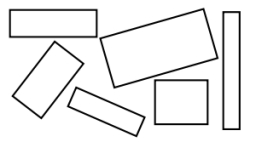Did you find an error or inaccuracy? Feel free to write us. Thank you!Tips to related online calculators
Line slope calculator is helpful for basic calculations in analytic geometry. The coordinates of two points in the plane calculate slope, normal and parametric line equation(s), slope, directional angle, direction vector, the length of the segment, intersections of the coordinate axes, etc.

#### You need to know the following knowledge to solve this word math problem:

We encourage you to watch this tutorial video on this math problem:

## Related math problems and questions:

• The coordinates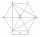The coordinates (5, 2) and (-6, 2) are vertices of a hexagon. Explain how to find the length of the segment formed by these endpoints. How long is the segment?
• Coordinates of the intersection of the diagonals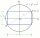In the rectangular coordinate system, a rectangle ABCD is drawn. The vertices of the rectangle are determined by these coordinates A = (2.2) B = (8.2) C = (8.6) D = (2.6) Find the coordinates of the intersection of the diagonals of the ABCD rectangle
• Vertices of a right triangleShow that the points D(2,1), E(4,0), F(5,7) are vertices of a right triangle.
• Rectangle 39Find the perimeter and area of the rectangular with vertices (-1, 4), (0,4), (0, -1), and (-4, 4)
• Coordinates of square verticesThe ABCD square has the center S [−3, −2] and the vertex A [1, −3]. Find the coordinates of the other vertices of the square.
• Coordinates hexagon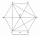The regular hexagon ABCDEF is given. Point A has coordinates [1; 3], and point D has coordinates [4; 7]. Calculate the sum of the coordinates of the center of its described circle.
• Find the 3Find the distance and midpoint between A(1,2) and B(5,5).
• BillboardRectangular billboard is 2.5 m long with a diagonal 2.8 m long. Calculate the perimeter and the content area of the billboard.
• Rectangle - parallelogram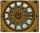It is given a rectangle that is circumscribed by a circle with a radius of 5 cm. The short side of the rectangle measures 6 cm. Calculate the perimeter of a parallelogram ABCD, whose vertices are the midpoints of the sides of the rectangle.
• SquarePoints A[9,9] and B[-4,1] are adjacent vertices of the square ABCD. Calculate the area of the square ABCD.
• Three pointsThree points A (-3;-5) B (9;-10) and C (2;k) . AB=AC What is value of k?
• What is 10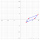What is this The area of a parallelogram that has vertices with the coordinates (0, 0), (4, 0),(5, 3), and (1, 3)?Show that the quadrilateral with vertices P1(0,1), P2(4,2) P3(3,6) P4(-5,4) has two right triangles.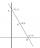Determine the area of the triangle given by line -7x+7y+63=0 and coordinate axes x and y.The area of the side of two cylinders is the same rectangle of 33 mm × 18 mm. Which cylinder has a larger volume and by how much?Determine the area of the rectangle where the length and width are in the ratio 5:2 and its length is 7.5 cm longer than its width. Determine also its length and its width.Circle is given by centre on S[-7; 10] and maximum chord 13 long. How many intersect points have circle with the coordinate axes?